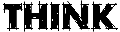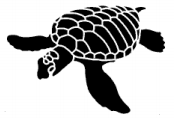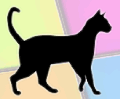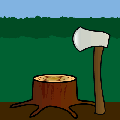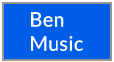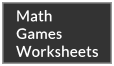Starters- More inside!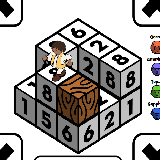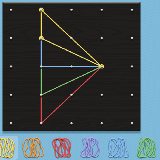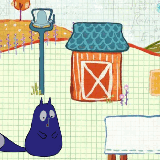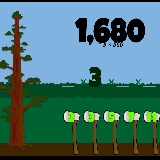Math Mine Diamonds Geoboard Math Snack Quest Stumped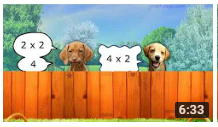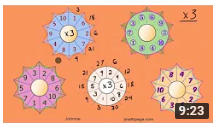Cat and Dog Multiply Multiplication Rings Square Donuts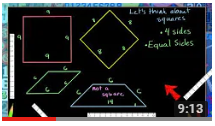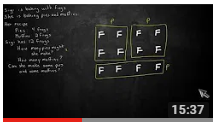Counting Cats Making Squares Frog Pie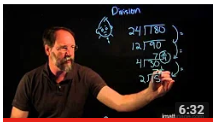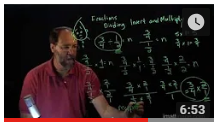Understanding Multiplication Division Simplified Fractions Invert and MultiplyMultiplication Practice